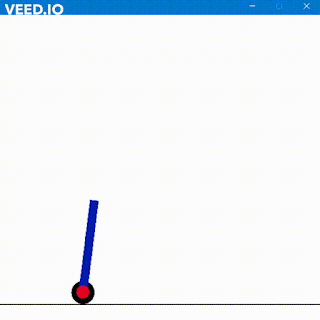## D FLIP FLOP

D flip flop stands for Delay Flip Flop. It acts as a buffer which delays the output by a clock cycle or as per desired. It’s a bistable multivibrator. D Flip Flop stores a single bit of data at a time.
It has two inputs. The first is the D. The second is the clock. Outputs are Q returning the state of machines and another is the complement of Q.

Truth Table

 D CLK Q Q’ 0 ↑ 0 1 1 ↑ 1 0 X 0 Q Q’ X 1 Q Q’

Assuming that we have considered the clock as positive edge for the D Flip Flop. When we apply 0 at the input D at the posedge the output is triggered and input is passed which here will be 0. At the negative edge of the same clock cycle the value of Q will retain and any change in the value of D won't affect the the value of Q. The complement of Q which is 1 here also remains in same state.
When we have 1 as input at D the output Q follows the same and gets triggered to 1. At the same moment the complement becomes 0.
The point here is setting Q to zero resets the D Flip Flop and setting Q to 1 sets the D flip flop.

Q -> 0 ( Reset when D = 0 )
Q -> 1 ( Set when D = 1 )
Whatever the FF captures the output gets the same captures data.
The following code is of Positive triggered FF. We can even do it for negative triggering. Al we have to do is to replace “posedge” to “negedge”

Code for D-FF (posedge)

module DFF(a,clk,b,bbar,reset);
input a,clk,reset;
output reg b;
output reg bbar;
initial begin
b = 0;
bbar = 1;
end
always @(posedge clk)begin
if(~reset)begin
b <= a;
bbar <= ~a;
end
else begin
b <= 1'bx;
bbar <= 1'bx;
end
end
endmodule

Code for D-FF (negedge)

module DFF(a,clk,b,bbar,reset);
input a,clk,reset;
output reg b;
output reg bbar;
initial begin
b = 0;
bbar = 1;
end
always @(negedge clk)begin
if(~reset)begin
b <= a;
bbar <= ~a;
end
else begin
b <= 1'bx;
bbar <= 1'bx;
end
end
endmodule

Code for Testbench of D-FF (Any edge)

module DFFtest;

// Inputs
reg a;
reg clk;
reg reset;
wire b;
wire bbar;
DFF uut (
.a(a),
.clk(clk),
.b(b),
.reset(reset),
.bbar(bbar)
);

initial begin
// Initialize Inputs
fork
a <= 0;
reset <= 0;
clk <= 0;
#10 a <= 1;
#15 reset <= 1;
#21 reset <= 0;
#40 a <= 0;
join
end
always #1 clk=!clk;
endmodule

Output Waveform

So Long